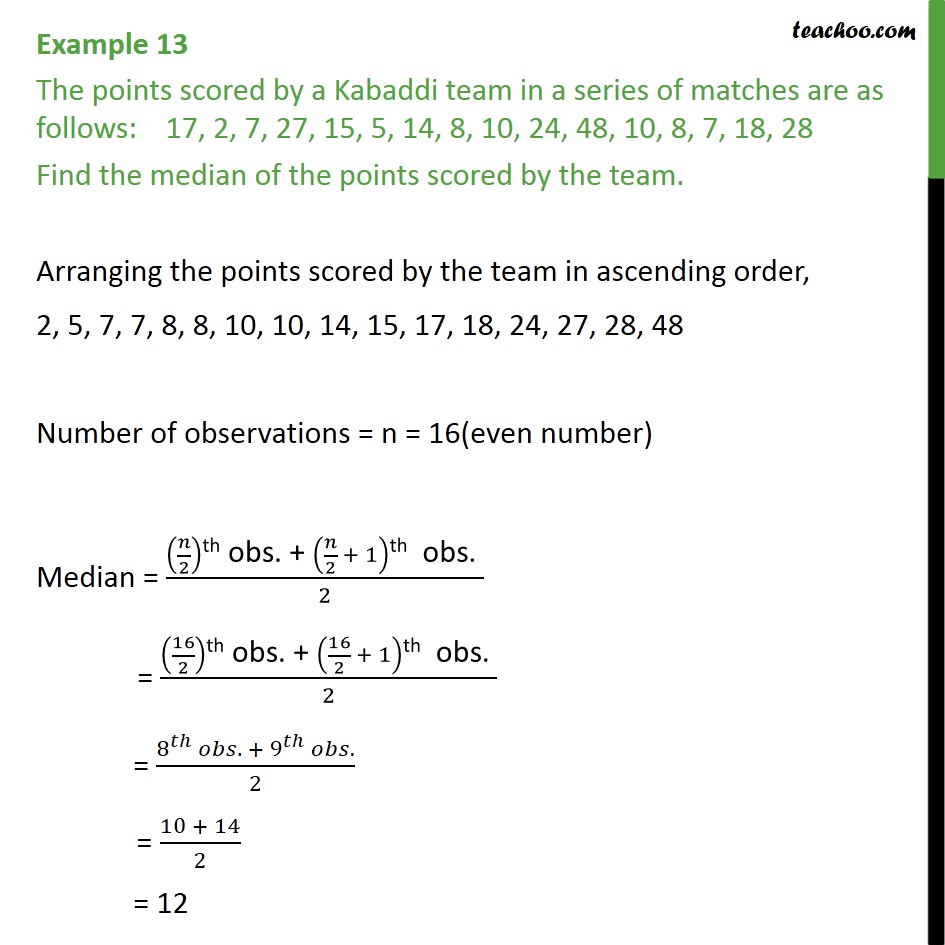Examples

Chapter 14 Class 9 Statistics
Serial order wiseGet live Maths 1-on-1 Classs - Class 6 to 12

### Transcript

Example 13 The points scored by a Kabaddi team in a series of matches are as follows: 17, 2, 7, 27, 15, 5, 14, 8, 10, 24, 48, 10, 8, 7, 18, 28 Find the median of the points scored by the team. Arranging the points scored by the team in ascending order, 2, 5, 7, 7, 8, 8, 10, 10, 14, 15, 17, 18, 24, 27, 28, 48 Number of observations = n = 16(even number) Median = ( /2)"th obs. + " ( /2 + 1)"th obs. " /2 = (16/2)"th obs. + " (16/2 + 1)"th obs. " /2 = (8^ . + 9^ .)/2 = (10 + 14)/2 = 12